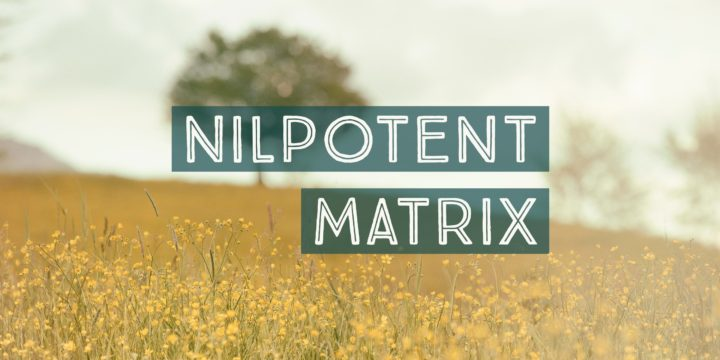# Sequences Satisfying Linear Recurrence Relation Form a Subspace## Problem 308

Let $V$ be a real vector space of all real sequences
$(a_i)_{i=1}^{\infty}=(a_1, a_2, \cdots).$ Let $U$ be the subset of $V$ defined by
$U=\{ (a_i)_{i=1}^{\infty} \in V \mid a_{k+2}-5a_{k+1}+3a_{k}=0, k=1, 2, \dots \}.$

Prove that $U$ is a subspace of $V$.Add to solve later

## Proof.

We prove the subspace criteria.

Subspace criteria

1. The zero vector in $V$ is in $U$.
2. For any two elements $(a_i)_{i=1}^{\infty}, (b_i)_{i=1}^{\infty}\in U$, we have $(a_i)_{i=1}^{\infty}+(b_i)_{i=1}^{\infty} \in U$.
3. For any scalar $c$ and any element $(a_i)_{i=1}^{\infty} \in U$, we have $c(a_i)_{i=1}^{\infty} \in U$.

Condition 1.

The zero vector in $V$ is the zero sequence $(0)=(0,0,0,\dots)$. Clearly, this sequence satisfies the recurrence relation $a_{k+2}-5a_{k+1}+3a_{k}=0$. Thus the zero vector $(0)\in U$, hence condition 1 is met.

Condition 2.

To check condition 2, take $(a_i)_{i=1}^{\infty}, (b_i)_{i=1}^{\infty}\in U$.
We want to show that the sum
$(a_i)_{i=1}^{\infty}+(b_i)_{i=1}^{\infty}=(a_i+b_i)_{i=1}^{\infty}$ is also in $U$.

Since $(a_i)_{i=1}^{\infty}, (b_i)_{i=1}^{\infty}\in U$, these sequences satisfy the recurrence relation
$a_{k+2}-5a_{k+1}+3a_{k}=0 \tag{*}$ and
$b_{k+2}-5b_{k+1}+3b_{k}=0$ for $k=1, 2, \dots$.
Using these two relations, we have
\begin{align*}
&(a_{k+2}+b_{k+2})-5(a_{k+1}+b_{k+1})+3(a_{k}+b_k)\\
&=(a_{k+2}-5a_{k+1}+3a_{k})+(b_{k+2}-5b_{k+1}+3b_{k})\\
&=0+0=0.
\end{align*}
Thus the sum $(a_i)_{i=1}^{\infty}+(b_i)_{i=1}^{\infty}=(a_i+b_i)_{i=1}^{\infty}$ satisfies the recurrence relation and it is also in $U$. Hence condition 2 is met.

Condition 3.

To check condition 3, take $(a_i)_{i=1}^{\infty} \in U$ and let $c\in \R$ be a scalar.
Then the sequence $(a_i)_{i=1}^{\infty}$ satisfies (*). Multiplying (*) by $c$, we have
$(ca_{k+2})-5(ca_{k+1})+3(ca_{k})=0.$ This implies that the scalar product $c(a_i)_{i=1}^{\infty}=(ca_i)_{i=1}^{\infty}$ satisfies the recurrence relation, and hence it is in $U$. Thus condition 3 is satisfied.

We have checked all subspace criteria, and thus $U$ is a subspace of the vector space $V$.

## Related Question.

This is the first problem of three problems about a linear recurrence relation and linear algebra.Add to solve later

### 3 Responses

1. 02/18/2017

[…] the post Sequences satisfying linear recurrence relation form a subspace for a proof that $U$ is a subspace of […]

2. 02/19/2017

[…] Sequences satisfying linear recurrence relation form a subspace […]

3. 03/02/2017

[…] Sequences satisfying linear recurrence relation form a subspace […]

This site uses Akismet to reduce spam. Learn how your comment data is processed.

###### More in Linear Algebra##### Example of a Nilpotent Matrix $A$ such that $A^2\neq O$ but $A^3=O$.

Find a nonzero $3\times 3$ matrix $A$ such that $A^2\neq O$ and $A^3=O$, where $O$ is the $3\times 3$ zero...

Close